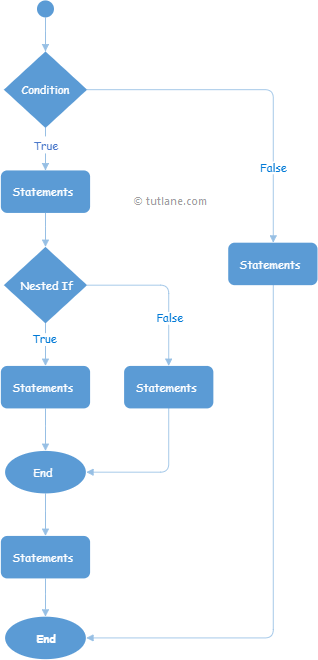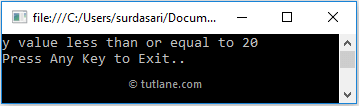# Visual Basic Nested If Else Statements

In Visual Basic, Nested If-Else statements are useful to include one if…else statement within another if…else statement to test one condition followed by another condition.

Generally, in Visual Basic, placing one if…else statement within another if…else statement is called a nested if…else statement.

## Syntax of Visual Basic Nested If-Else Statement

Following is the syntax of defining the nested if…else statement in a visual basic programming language.

If condition Then
If nested_condition_1 Then
// Statements to Execute
Else
// Statements to Execute
End If
Else
If nested_condition_2 Then
// Statements to Execute
Else
// Statements to Execute
End If
End If

If you observe the above Visual Basic nested if-else statement syntax, we defined one if…else statement within another if…else condition to perform one condition followed by another condition.

In the nested If-Else statement, the defined If condition returns true, then it will enter into the body of condition and perform another if…else condition checking based on our requirements.

## Visual Basic Nested If-Else Statement Flow Chart

Following is the flow chart diagram, representing the process flow of the nested-if-else statement in a visual basic programming language.If you observe the above Visual Basic nested if-else statements flow chart, when the defined condition is true, then another if…else condition execution will happen and perform the required operations. Same way, other nested if…else statements also will be executed based on our requirements.

## Visual Basic Nested If-Else Statement Example

Following is the example of defining a nested If-Else statement in Visual Basic programming language to execute the block of code or statements followed by another block of code based on our requirements.

Module Module1
Sub Main()
Dim x As Integer = 5, y As Integer = 20
If x > y Then
If x >= 10 Then
Console.WriteLine("x value greater than or equal to 10")
Else
Console.WriteLine("x value less than 10")
End If
Else
If y <= 20 Then
Console.WriteLine("y value less than or equal to 20")
Else
Console.WriteLine("y value greater than 20")
End If
End If
Console.WriteLine("Press Enter Key to Exit..")
End Sub
End Module

If you observe the above example, we defined nested if-else statements to execute one condition followed by another condition based on our requirements.

When we execute the above Visual Basic program, we will get the result as shown below.If you observe the above result, the nested if-else statements have been executed based on the conditions we defined and printed the required statement in the console window.

This is how we can use the nested if-else statements in the Visual Basic programming language to execute the block of code or statements followed by another block of code or statements based on our requirements.